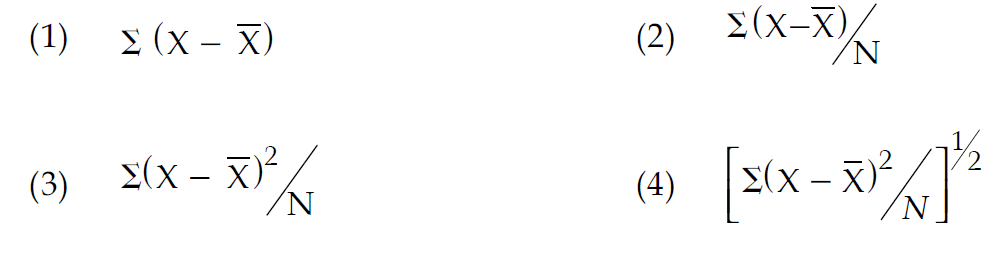# GEOGRAPHICAL TECHNIQUES PYQS JUNE & DEC 2015 NTA UGC NET

GEOGRAPHICAL TECHNIQUES (CARTOGRAPHY & STATS) PYQS JUNE & DEC 2015 NTA UGC NET

GEOGRAPHICAL TECHNIQUES PYQS DEC 2015 NTA UGC NET, GEOGRAPHICAL TECHNIQUES  NTA UGC NET DEC 2015,GEOGRAPHICAL TECHNIQUES  PYQS UGC NET JUNE 2015, GEOGRAPHICAL TECHNIQUES  PYQS DEC 2015 NTA UGC NET PYQS GEOGRAPHY, GEOGRAPHICAL TECHNIQUES  PYQS UGC NET, PRACTICAL GEOGRAPHY PYQS NTA UGC NET, CARTOGRAPHY PYQS NTAUGC NET, STATS PYQS NTAUGC NET, GEOGRAPHY PYQS UGC NET,

(PDF) For download PDF file of 2015 UGC NET – go to the bottom of this post.

Q.1. Which one of the following cartographic techniques is suitable for measuring spatial association among different attributes of the regional economy? (UGC NET JUNE 2015)

(1) Bar diagram

(2) Pie diagram

(3) Choropleth

(4) Isopleth

Q.2. Match List-I with List-II and select the correct answer from the codes given below: (UGC NET JUNE 2015)

List-I (Method)

(a) Isopleth

(b) Dot maps

(c) Scatter diagrams

(d) Star diagrams

List-II (uses)

(i) Natural groupings

(ii) Distribution of population

(iii) Wind direction

(iv) Changes

Codes:

 (a) (b) (c) (d) (1) (iv) (ii) (iii) (i) (2) (ii) (iv) (iii) (i) (3) (iv) (ii) (i) (iii) (4) (ii) (iv) (i) (iii)

##### Q.3. Which one of the following measures of central tendency is used to indicate the ‘centre of population’?  (UGC NET JUNE 2015)

(1) Median

(2) Mode

(3) Mean

(4) Harmonic mean

##### Q.4. Which one of the following terms shows variance of a particular distribution? (UGC NET JUNE 2015)Q.5. Which one of the following is correct? (UGC NET JUNE 2015)

(1) Arithmetic mean is a numerical value

(2) Arithmetic mean is not affected by the variability in the data sets

(3) Arithmetic mean is always a positive number

(4) Arithmetic mean is not useful for any further statistical analysis of the data

Q.6. Which one of the following central tendencies is the appropriate method for the study of dispersion? (UGC NET JUNE 2015)

(a) Arithmetic mean

(b) Median

(c) Mode

(d) Geometric mean

Q.7. Which one of the following data can be represented by means of Choropleth map? (UGC NET DEC 2015)

(1) Location Specific

(2) Point Specific

(3) Area specific

(4) Line Specific

Q.8. Match List-I with List – II and select the correct answer from the codes given below: (UGC NET DEC 2015)

List – I (Aspect)

(a) Rural and Urban Population

(b) population density

(c) Relative relief

List – II (Diagram)

(i) Comparative Bars

(ii) Isolines

(iii) Dots and Spheres

(iv) Choropleth

Codes:

(a) (b) (c) (d)

(1) (iv) (iii) (i) (ii)

(2) (i) (ii) (iii) (iv)

(3) (ii) (i) (iv) (iii)

(4) (iii) (iv) (ii) (i)

Q.9. Which of the following diagrams are called Pie-diagrams? (UGC NET DEC 2015)

(1) Pictorial diagrams

(2) Circle and sector diagrams

(3) Ring diagrams

(4) Sphere diagrams

Q.10. Lorenz curve is used to find out which of the following? (UGC NET DEC 2015)

(1) Relative concentration of a phenomenon

(2) Absolute concentration of a phenomenon

(3) Both relative and absolute concentration of a phenomenon

(4) Probability of dispersion of a phenomenon

Q.11. Match List – I with List – II and select the correct answer from the codes given below Table (UGC NET DEC 2015)

List – I (Spatial Pattern)

(a) Perfect Uniform

(b) Tending to Cluster

(c) Tending to Random

(d) Perfect Cluster

List – II (Rn. Statistics)

(i) 1.20

(ii) 2.14

(iii) 0.00

(iv) 0.60

Codes:

(a)   (b)   (c)   (d)

(1) (iii)  (i)   (iv)   (ii)

(2) (i)   (iii)  (ii)   (iv)

(3) (ii)  (iv)   (i)   (iii)

(4) (iv)  (ii)  (iii)   (i)

Q.12. Which one of the following codes is correct to depict lesser degree of significance of given distributions? (UGC NET DEC 2015)Codes:

(1) (a) and (b)

(2) (a) and (d)

(3) (b) and (d)

(4) (b) and (c)